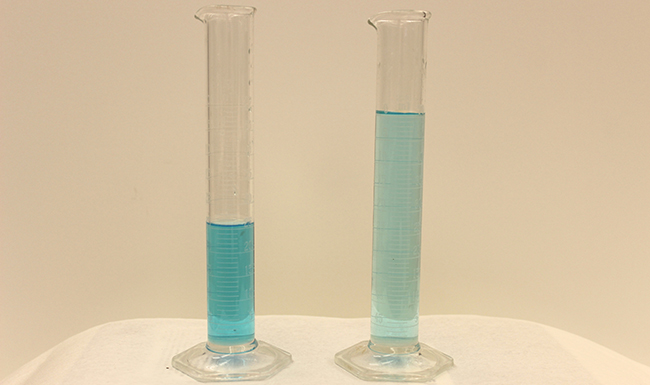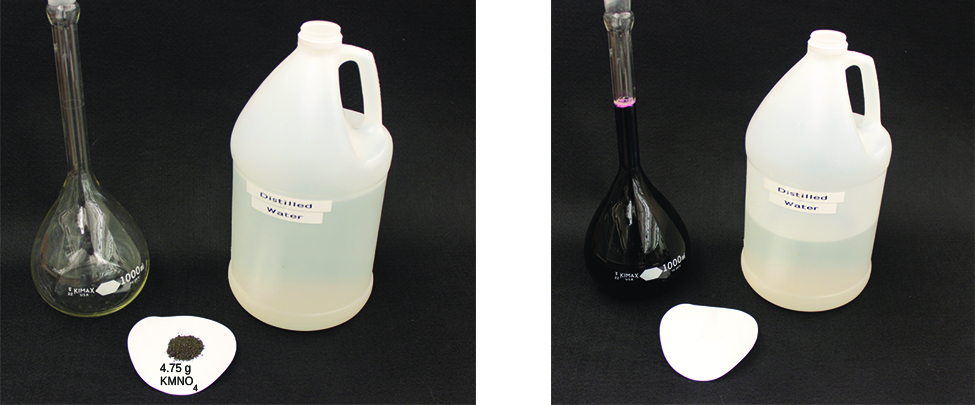# 3.3 Molarity

 Page 3 / 14

## Dilution of solutions

Dilution is the process whereby the concentration of a solution is lessened by the addition of solvent. For example, we might say that a glass of iced tea becomes increasingly diluted as the ice melts. The water from the melting ice increases the volume of the solvent (water) and the overall volume of the solution (iced tea), thereby reducing the relative concentrations of the solutes that give the beverage its taste ( [link] ).Both solutions contain the same mass of copper nitrate. The solution on the right is more dilute because the copper nitrate is dissolved in more solvent. (credit: Mark Ott)

Dilution is also a common means of preparing solutions of a desired concentration. By adding solvent to a measured portion of a more concentrated stock solution , we can achieve a particular concentration. For example, commercial pesticides are typically sold as solutions in which the active ingredients are far more concentrated than is appropriate for their application. Before they can be used on crops, the pesticides must be diluted. This is also a very common practice for the preparation of a number of common laboratory reagents ( [link] ).A solution of KMnO4 is prepared by mixing water with 4.74 g of KMnO4 in a flask. (credit: modification of work by Mark Ott)

A simple mathematical relationship can be used to relate the volumes and concentrations of a solution before and after the dilution process. According to the definition of molarity, the molar amount of solute in a solution is equal to the product of the solution’s molarity and its volume in liters:

$n=ML$

Expressions like these may be written for a solution before and after it is diluted:

${n}_{1}={M}_{1}{L}_{1}$
${n}_{2}={M}_{2}{L}_{2}$

where the subscripts “1” and “2” refer to the solution before and after the dilution, respectively. Since the dilution process does not change the amount of solute in the solution, n 1 = n 2 . Thus, these two equations may be set equal to one another:

${M}_{1}{L}_{1}={M}_{2}{L}_{2}$

This relation is commonly referred to as the dilution equation. Although we derived this equation using molarity as the unit of concentration and liters as the unit of volume, other units of concentration and volume may be used, so long as the units properly cancel per the factor-label method. Reflecting this versatility, the dilution equation is often written in the more general form:

${C}_{1}{V}_{1}={C}_{2}{V}_{2}$

where C and V are concentration and volume, respectively.

## Determining the concentration of a diluted solution

If 0.850 L of a 5.00- M solution of copper nitrate, Cu(NO 3 ) 2 , is diluted to a volume of 1.80 L by the addition of water, what is the molarity of the diluted solution?

## Solution

We are given the volume and concentration of a stock solution, V 1 and C 1 , and the volume of the resultant diluted solution, V 2 . We need to find the concentration of the diluted solution, C 2 . We thus rearrange the dilution equation in order to isolate C 2 :

$\begin{array}{c}{C}_{1}{V}_{1}={C}_{2}{V}_{2}\\ \\ {C}_{2}=\phantom{\rule{0.2em}{0ex}}\frac{{C}_{1}{V}_{1}}{{V}_{2}}\phantom{\rule{0.2em}{0ex}}\end{array}$

Since the stock solution is being diluted by more than two-fold (volume is increased from 0.85 L to 1.80 L), we would expect the diluted solution’s concentration to be less than one-half 5 M . We will compare this ballpark estimate to the calculated result to check for any gross errors in computation (for example, such as an improper substitution of the given quantities). Substituting the given values for the terms on the right side of this equation yields:

${C}_{2}=\phantom{\rule{0.2em}{0ex}}\frac{0.850\phantom{\rule{0.2em}{0ex}}\text{L}\phantom{\rule{0.2em}{0ex}}×\phantom{\rule{0.2em}{0ex}}5.00\phantom{\rule{0.2em}{0ex}}\frac{\text{mol}}{\text{L}}\phantom{\rule{0.2em}{0ex}}}{1.80 L}\phantom{\rule{0.2em}{0ex}}=2.36\phantom{\rule{0.2em}{0ex}}M$

This result compares well to our ballpark estimate (it’s a bit less than one-half the stock concentration, 5 M ).

What is the concentration of the solution that results from diluting 25.0 mL of a 2.04- M solution of CH 3 OH to 500.0 mL?

0.102 M CH 3 OH

How does an element differ from a compound? How are they similar?
an element is an indivisible particles that can take part in a reaction and consist of smaller or tiny particles i.e proton, neutrons and electron while a compound is when two or more element chemically combine together. They are similar when they are homogeneous compound. they take the same rxn.
Yusuf
How to get the Lewis formula of SeCl+3
hi,I'm new here can I join the conversation
EZEA
what is the structural formula for starch
Starch is a mixture (of chemicals) of amylose and amylopectin. Both are macromolecules and polymers. You can search on wikipedia.
Abdelkarim
what is the roles of filter bed
Fathmat
what is the roles of Alu m
Fathmat
what is the roles of chlorine
Fathmat
Roles can be classified or correlate it to different areas: For example: Chlorine can be used in reactions (in industry) to manufacture HCl, which then can be used for other things. Or in swimming pools to kill bacteria. Or as a component in compounds with pharmaceutical roles (drugs). For Al:
Abdelkarim
Its dentisty value is suitable to be used in alloys (mixture of metals) in aircraft bodies. Also, Aluminium foils, Tin cans,.. Some of them are also in Al overhead cables in streets and long roads.
Abdelkarim
what is chemistry
Maxamed
what is the meaning of exceedingly
it is an adverb which means extremely
Rohini
what is atomic chemistry?
Lewis structure for no3
Lewis structure for no3
what is weak acid
It is an acid which partially ionises in water.
Abdelkarim
what is incandescence
Clifton
what makes it glow
Clifton
why is it red, irange and yellow in color
Clifton
hello am new here and I want to join you
Aliyu
hello
Clifton
hi
Aliyu
too
Gillian
hello i am new here please i want to join this group
Paul
Hi, I'm also new here
Salaudeen
Hi
Keeya
hello guys !!
Sourav
what is pressure?
The force applied to suction Area of the body
Ahmed
Matter composed of exceedingly small paticle called atom.
Yushao
questions related to metals
occurrence and preparation of the representatives metals
Regina
list the 20, periodic table and their symbols
hydrogen:h helium;he lithium:l beryllium:be Boron:b Carbon;C Nitrogen:n Oxygen:O FLUORINE:f Neon:n Sodium:s Magnesium:mg Aluminum:a Silicon:s Phosphorus:p Sulphur:s Chlorine:c Argon;a Potassium:p Calcium:c
Benita
Hydrogen, helium, lithium, beryllium, boron, carbon, nitrogen, oxygen, fluorine, neon, sodium, magnesium, aluminium, silicon, phosphorus, sulphur, chlorine, argon, potassium, calcium
Cudjoe
what is a solute
Any substance that is disolved in a liqid solvent to create a solution
Fifa
sorry liquid
Fifa
it's a liquid substance
Fathmat
hello group
Ayomide
is the substance that dissolves in the solvent
Amos
so is HCl ionic compound
No, covalent compound ➡️ molecule. As both H and Cl are non-metals and and form covalent bind by sharing valence e-. But can fully ionice in water forming H+ (a proton, a reason for acidity) and Cl- (anion =Chloride) Hydrogen Chloride is a gas at room; Hydrochloric acid = HCl (aq), dissolved in w
Abdelkarim
Form covalenr bond*
Abdelkarim
The question marks are an emoji in the first sentence is an unread emoji. HCl Covalent compund -> molecule
Abdelkarim
Hi.
Queen
Hi
Calvin
Yh
Cudjoe
yes
Amos
what is chemistry
is the study of composition of substances and the way they behave under different conditions
Amos
how do calculate n1 though n6 any help on understanding the concept
Clifton
where can I get the test bank or mcqs ? any idea ?
what are the types of intermolecular forces between organic compounds
Intermolecular forces exist between molecules of different units like van der waal force, hydrogen bonds
Salaudeen
What is chemistry
scientific study of structure of substances and of the way that they react with other substances
Haider
Thanks
khausar
welcome
Haider
Hi
khausar
hi 2
Haider
whr u from
Haider
are u writing GCE
Equin
Cameroon and u
Equin
hello
DanielByByBy OpenStaxBy CB BiernBy Monty HartfieldBy Abishek DevarajBy OpenStaxBy OpenStaxBy OpenStaxBy OpenStaxBy OpenStaxBy Madison Christian